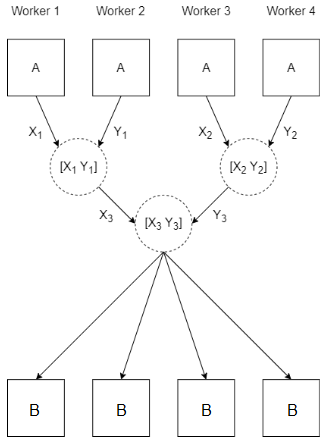# gcat

Concatenate arrays on `spmd` workers

## Syntax

``B = gcat(A)``
``B = gcat(A,dim)``
``B = gcat(A,dim,destination)``

## Description

example

````B = gcat(A)` horizontally concatenates the array `A` defined on each worker running an `spmd` block or communicating job.When you use `parfor`, `parfeval`, or `parfevalOnAll` to run code on a parallel pool, the workers are independent and do not communicate with each other. If you use `gcat` on these workers, the result is the same as `gcat` on a client.You can use `gcat` only if the array `A` defined on each worker can be concatenated along the second dimension.`gcat` concatenates the arrays along the second dimension, then stores the result on all workers. The value of `A` is taken from each worker and concatenated in `labindex` order. For example, `gcat(labindex)` returns `1:numlabs`.If `numlabs` is equal to `1`, `B` is equal to `A`.```
````B = gcat(A,dim)` concatenates the array `A` defined on each worker along dimension `dim`. You can use `gcat` only if the array `A` concatenates along dimension `dim` when all arrays have compatible sizes (the lengths of the dimensions match except for the operating dimension dim).```
````B = gcat(A,dim,destination)` concatenates the array `A` defined on each worker and stores the result on only one worker.```

## Examples

collapse all

This example shows how to use `gcat` to concatenate a scalar held on each worker in an `spmd` block.

Create a parallel pool with 4 workers.

`parpool(4);`

Create an `spmd` block. By default, each worker in the pool runs the `spmd` block. Store the value returned by `labindex` on each worker as `A`, then use `gcat` to take the value of `A` defined on each worker and concatenate them.

When you use `gcat`, the result is stored by default on every worker. On the client, the result is a `Composite` array. To get the result, index into the `Composite` array.

```spmd B = gcat(labindex); end B{1}```
```ans = 1 2 3 4```

## Input Arguments

collapse all

Input array, specified as a scalar, vector, matrix, multidimensional array, table, timetable, or any MATLAB variable that supports concatenation.

Example: `A = magic(3)`

Dimension to operate along, specified as a positive integer scalar. For example, if `A` is a 2-by-2 matrix on all workers, then `gcat(A,1)` concatenates vertically creating a `2`*`numlabs`-by-2 matrix. `gcat(A,2)` concatenates horizontally creating a 2-by-`2`*`numlabs` matrix.

The default value is `2`.

`dim` must be either 1 or 2 for table or timetable input.

Index of destination worker, specified as a positive integer scalar. The value must be less than or equal to the value given returned when you use `numlabs` in the current `spmd` block or communicating job. When specified, the result `B` from running `B = gcat(A,dim,destination)` is only stored on one worker. The array `B` is still defined on all workers that run `gcat`. On the worker with `labindex` equal to `destination`, `C` is the result of the concatenation. On all other workers, `C` is `[]`.

## Output Arguments

collapse all

Output array, returned as any MATLAB variable.

If you specify `destination`, `B` is the result of the concatenation on the worker with `labindex` equal to `destination`. On all other workers, `B` is `[]`.

## Algorithms

When you use `gcat(A)`, the value of `A` on each worker is concatenated with the value on other workers.## Version History

Introduced in R2006b